# How to use the EXP function in Excel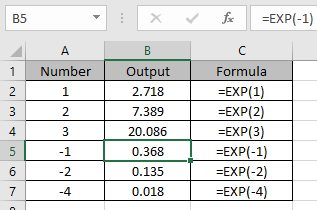In this article, we will learn How to use the EXP Function in Excel.

What are exponents in Excel ?

In simple words, the exponent of a number is, the number of times the number gets multiplied.

= 23  (In Mathematics) = 8

= 2 ^ 3 (In Excel) =  8

Caret ( ^ ) sign is used to take the POWER of a number. To use this method first start with equals ( = ) sign  and then use the caret ( ^ ) sign between the numbers, to Power.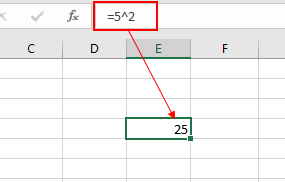As shown in the above snapshot the 2 Power of 5 comes out to be 25, which is 5 x 5 equals 25.

There are 3 ways to use Power of a number in Excel.

1. Use the multiply character ( ^ )
2. Excel POWER function
3. Use the EXP function only to get powers of e (e = 2.7128..).

e is a euler number invented by John Napier. It is an irrational number written as e = 2.718281828459... . This is generally used as the base of the natural logarithmic function. This is a very valuable mathematical number and widely used in mathematics. Let's learn more about EXP function syntax and an example to illustrate the function usage.

EXP Function in Excel

EXP function returns a number when number 2.7128… which is e gets the power of number n. For example when =e * e * e = e^3 or can be extracted using the below function =EXP(3).

Syntax:

 =EXP(number)

number : any numerical value

Example :

All of these might be confusing to understand. Let's understand how to use the function using an example. Here we have some numbers to give input for EXP function.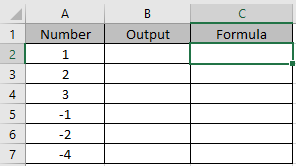Use the formula:

 =EXP(1)

The value returned here will the value of e.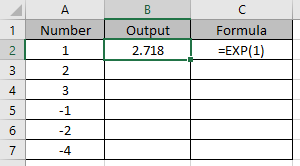Use the formula in other cells to get the powers of irrational constant “e”.As you can see, the function returns the value of e and its power. LN function is a type of mathematical function which also uses irrational number as base. Let's check out below

LN function in Excel

Here we have numbers in Column A. we need to find the LN of these numbers.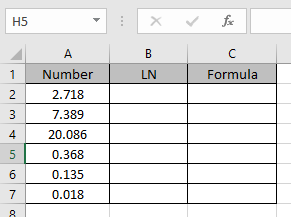These numbers are generated using EXP functions as we can’t get the constant value of “e” in Excel.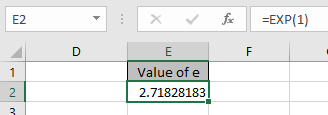Use the formula

 =LN(A2)

Here LN returns the Logarithmic value in A2 at base “e”.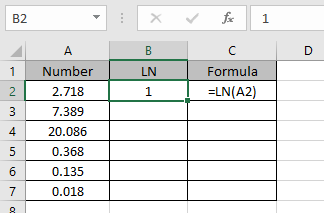e1= 2.7128..

1 = loge(e)

The above stated equation stats that LN(e) = 1

Now we will apply the formula to other cells to get the LN of all numbers.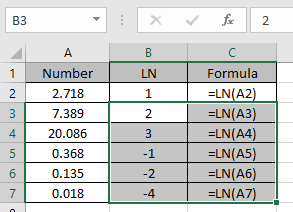As you can see LN function returns the logarithmic of the numbers at base e.

Here are all the observational notes using the EXP function in Excel
Notes :

1. Any non numeric value given as input will return as error by function
2. You can feed the arguments to the function directly or using the cell reference as explained in the example.

Hope this article about How to use the EXP function in Excel is explanatory. Find more articles on mathematical calculations values and related Excel formulas here. If you liked our blogs, share it with your friends on Facebook. And also you can follow us on Twitter and Facebook. We would love to hear from you, do let us know how we can improve, complement or innovate our work and make it better for you. Write to us at info@exceltip.com.

Related Articles :

How to use the ROUND function in Excel | rounds off the given number to the nearest num_digit decimal using the ROUND function in Excel

How to use the ROUNDUP function in Excel | rounds up the given number to the nearest num_digit decimal using the ROUNDUP function in Excel

How to use the ROUNDDOWN function in Excel | rounds down the given number to the nearest num_digit decimal using the ROUNDDOWN function in Excel

How to use Excel F.TEST Function in Excel : The F.TEST Function is used to calculate F statistic of two samples in excel internally and returns the two tailed probability of the F statistic under Null Hypothesis.

How To Use Excel T.TEST Function in Excel : The T.TEST function is used to determine the confidence of an analysis in Excel. Mathematically, it is used to know if the mean of the two samples are equal or not. T.TEST function is used to accept or reject the null hypothesis.

How to use the DEVSQ Function in Excel : DEVSQ function is a built-in statistical function to calculate the sum of squared deviations from the mean or average of the given distribution provided.

How to calculate Standard Deviation in Excel : To calculate the standard deviation we have different functions in Excel. The standard deviation is the square root of the variance value but It tells more about the dataset than variance.

How to use the VAR function in Excel : Calculate the variance for the sample dataset in excel using the VAR function in Excel.

Popular Articles :

How to use the IF Function in Excel : The IF statement in Excel checks the condition and returns a specific value if the condition is TRUE or returns another specific value if FALSE.

How to use the VLOOKUP Function in Excel : This is one of the most used and popular functions of excel that is used to lookup value from different ranges and sheets.

How to use the SUMIF Function in Excel : This is another dashboard essential function. This helps you sum up values on specific conditions.

How to use the COUNTIF Function in Excel : Count values with conditions using this amazing function. You don't need to filter your data to count specific values. Countif function is essential to prepare your dashboard.

Terms and Conditions of use

The applications/code on this site are distributed as is and without warranties or liability. In no event shall the owner of the copyrights, or the authors of the applications/code be liable for any loss of profit, any problems or any damage resulting from the use or evaluation of the applications/code.